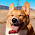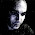## Monday, May 3, 2010

### Abstract Types vs Parameter

This topic (and the next) are intended to discuss abstract types. A class/trait with an abstract type is quite similar to a class/trait type parameter. For example:

```trait C[A] {   def get : A   def doit(a:A):A } trait C2 {   type A   def get : A   def doit(a:A):A } ```
Both implementations have similar properties. However they are NOT the same. At first I thought that I could used them inter-changeably. However, consider the following examples:

```//compiles def p(c:C[Int]) = c.doit(c.get) // doesn't compile def p2(c:C2) = c.doit(c.get) ```
So why doesn't p2 compile? Because it returns A. From the signature of p2 it is impossible to know what p2 returns. There are several ways to fix this problem. One make the method return Unit:

```// compiles because the internals of C2 does not leak out def p(c:C2):Unit = c.doit(c.get) ```
Another fix would be to change doit to return Unit or an explicit return value like Int

```trait C2 {   type A   def get : A   def doit(a:A):Int } // compiles correctly def p(c:C2) = c.doit(c.get) ```
A second difference between parameterized types and types with abstract type values is illustrated below:

```trait C2 {    type A    def get : A } scala> var c : C2 = new C2 {       | type A = Int      | def get = 3      | } c: C2 = \$anon\$1@11a40fff // what is the type of result if at compile time the // value of c is not known scala> var result = c.get result: C2#A = 3 scala> c = new C2 {            |    type A = String      |    def get = "hi"      | } c: C2 = \$anon\$1@5f154718 // crazy eh :) the variable can be anything but does not // have type Any so you cannot assign arbitrary values scala> result = c.get result: C2#A = hi scala> result.isInstanceOf[String] res0: Boolean = true // while the dynamic type of result is a string the // static type is not so you cannot assign a string to result scala> result = "4" < console> :8: error: type mismatch;  found   : java.lang.String("4")  required: C2#A        result = "4"                 ^ ```
The obvious question is what use are abstract types. I don't claim to know them all but the main point is that they do not expose the internal implementation details to the world. The famous cake pattern is one such example usage of abstract types.

I read the following as well (wish I could remember where):

Abstract types are good when extending and there will be concrete subclasses. Param type good for when a type is useful without extension but can handle several types.

A simpler example is examined here. It is loosely based on a real world usecase.
The example below is contrived so that it is smaller than the actual usecase, so consider the design and not the fact that the example could be easier done with other examples. In the real scenario this design reduced the lines of duplicated code from around 500 to 10.

The example below shows how a Traversable like object can be created from InputStreams and Readers. The important aspect is that the type signature of Foreach does not leak information about the implementation. Users of a Foreach object don't care whether it is backed onto an InputStream or Reader. They just care about the type of object contained.

I am leaving this already long post here. The next post will investigate different ways you can get in trouble trying to implement using abstract types.

```import java.io.{InputStream, Reader, ByteArrayInputStream, StringReader} import java.net.URL object Foreach {   def fromStream(s: => InputStream) = new Foreach[Int] {     type I = InputStream     def source = new Source {       def in = s       def next(_in : InputStream) = _in.read match {         case -1 => None         case i => Some(i)       }     }   }      def fromReader(s: => Reader) = new Foreach[Char] {     type I = Reader     def source = new Source {       def in = s       def next(_in : Reader) = _in.read match {         case -1 => None         case i => Some(i.toChar)       }     }   }         def fromInputAndFunction[A](s: => InputStream, f: Int => A) = new Foreach[A] {     type I = InputStream     def source = new Source {       def in = s       def next(_in : InputStream) = _in.read match {         case -1 => None         case i => Some(f(i))       }     }   }       } trait Foreach[A] {   type I <: java.io.Closeable   trait Source {     def in : I     def next(in : I) : Option[A]   }   def source : Source      def foreach[U](f : A => U) : Unit = {     val s = source.in     try {       def processNext : Unit = source.next(s) match {         case None =>            ()         case Some(value) =>            f(value)           processNext       }              processNext     } finally {       // correctly handle exceptions       s.close     }   } } object Test {   def main(args : Array[String]) = {     val data = "Hello World"     val bytes = data.toArray.map { _.toByte }     import Foreach._     fromStream(new ByteArrayInputStream(bytes)).foreach {a => print(a.toChar)}          println     fromReader(new StringReader(data)) foreach print          println          fromInputAndFunction(new ByteArrayInputStream(bytes), i => i.toChar) foreach print          println   } } ```

1."while the dynamic type of result is a string the dynamic type is not so you cannot assign a string to result"

Didn't you mean:
"while the dynamic type of result is a string the *static* type is not so you cannot assign a string to result" ?

2.thanks. I've updated it

3.Abstract types can also be used to implement recursion of types in some situations where type parameters cannot.

4.5.6.1.